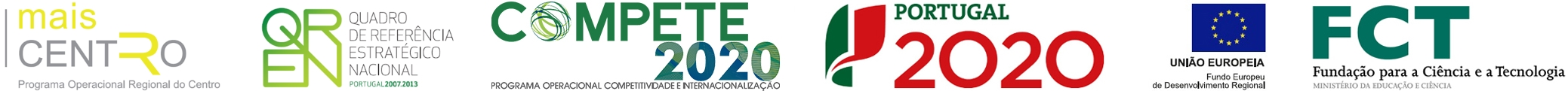Magnetic entropy change of Pr1-xCaxMnO3 manganites (0.2 <= x <= 0.95)
 authors Reis, MS; Amaral, VS; Araujo, JP; Tavares, PB; Gomes, AM; Oliveira, IS nationality International journal PHYSICAL REVIEW B keywords COLOSSAL MAGNETORESISTANCE; MANGANESE OXIDES; PR-0.6(CA1-XSRX)(0.4)MNO3 0-LESS-THAN-OR-EQUAL-TO-X-LESS-THAN-OR-EQUAL-TO-1; MAGNETOCALORIC PROPERTIES; PEROVSKITE MANGANITES; TRANSPORT-PROPERTIES; NEUTRON-DIFFRACTION; PHASE-TRANSITION; CHARGE ORDER; PR0.7CA0.3MNO3 abstract In the present work we analyze the magnetic entropy change Delta S-M of the Pr1-xCaxMnO3 manganites, for a wide range of Ca concentrations (0.20 <= x <= 0.95). The results for the samples with 0.20 < x <= 0.30 present the usual behavior expected for ferromagnetic systems, peaking at the Curie temperature T-C. In contrast, for the charge-ordered antiferromagnetic samples (0.30 < x < 0.90), an anomalous magnetic entropy change starts around the charge-ordering temperature T-CO, persisting for lower values of temperature. This effect is associated to a positive contribution to the magnetic entropy change due to the charge-ordering Delta S-CO, which is superimposed to the negative contribution from the spin-ordering Delta S-spin; that is described using a mean-field approximation. Supposing these contributions, we could also appraise Delta S-CO(max) as a function of Ca content, which vanishes for the limits x similar to 0.30 and 0.90 and presents a deep minimum around x similar to 0.50, with two maxima at x similar to 0.35 and 0.65. We conclude that for x>0.65 only the magnetic order governs the charge ordering, contrarily to x < 0.65, where there are more than one mechanism ruling the charge ordering. Moreover, for the samples with phase coexistence (0.30 < x <= 0.40), we found extremely large values for the magnetic entropy change at low temperatures. Finally, for x>0.90, we found usual magnetic entropy change curves, peaking at the Neel temperature T-N. publisher AMERICAN PHYSICAL SOC issn 1098-0121 year published 2005 volume 71 issue 14 digital object identifier (doi) 10.1103/PhysRevB.71.144413 web of science category Physics, Condensed Matter subject category Physics unique article identifier WOS:000228758700051
ciceco authors
impact metrics
 journal analysis (jcr 2019): journal impact factor 3.575 5 year journal impact factor 3.511 category normalized journal impact factor percentile 70.187
 dimensions (citation analysis): altmetrics (social interaction):